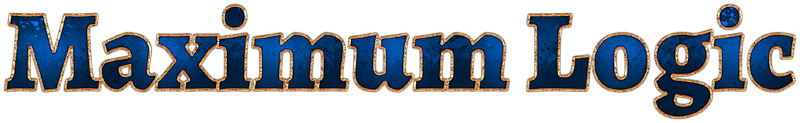### Solution

The logic operators used here are the same as in several logic-based KTANE modules, including Logic, Superlogic and Boolean Venn Diagram.

The provided logic statements are only consistent if the truth assignment is as follows.

W = ¬(YM)

P = ¬(IB)

C = ¬JU

H = ¬(GP)

L = NH

O = B → ¬D

I = ¬OM

S = L ∧ ¬Y

B = ¬WS

T = UB

Q = ¬NV

U = B | ¬N

J = ¬(YS)

F = ¬(RE)

R = V | W

K = OD

X = J ∨ ¬G

A = R ⊻ ¬C

N = TK

D = YR

G = ¬QD

V = ¬WY

E = YU

M = ¬(AC)

Y = L ∧ ¬W

Z = ¬(J | L)

The solution word consists of the letters whose value is true, in the order given.

COURAGE